Latest Banking jobs   »   Reasoning Ability Quiz For IBPS RRB...

# Reasoning Ability Quiz For IBPS RRB PO, Clerk Prelims 2021- 15th May

Directions (1-5): Study the following information carefully and answer the questions given below:
Eight students A, B, C, D, E, F, G and H are giving a competitive exam in the months of August, September, October and November but not necessarily in the same order. In each month, they give their exam on either 15th or 20th of the given month. H gives exam on 15th of any of the month which has 31 days. Only one student gives exam after F. Only two students give exam between F and B. Only one student gives exam between B and C. A give exam immediately after G. D does not give exam on the last day and doesn’t give exam in the month which has 30 days. G does not give exam after D.

Q1. On which month H gives his exam?
(a) August
(b) September
(c) October
(d) November
(e) Can’t be determine

Q2. Which of the combination is true about B?
(a) November-15
(b) September-20
(c) October-15
(d) August-20
(e) None of these

Q3. How many students give their exam after D?
(a) One
(b) Two
(c) Four
(d) Three
(e) None

Q4. Who among the following gives exam before D but after A?
(a) F
(b) E
(c) A
(d) B
(e) None of these

Q5. Who among the following gives their exam on 20th November?
(a) G
(b) E
(c) F
(d) H
(e) D

Q6. A person starts walking in the east direction from point A and walks 12m to reach at point B. From Point B he takes two consecutive right turn and walks 10m and 24m to reach at point C. Finally, he starts walking in north direction from point C and walks 5m to reach at point D. What is the shortest distance and direction of point D point with respect to point A?
(a) 20m, South-west
(b) 15m, North-east
(c) 13m, South-west
(d) 13m, North-west
(e) None of these

Directions (7-9): Study the following information carefully and answer the questions given below:
Point A is 8m north of point B. Point E is 5m south of point F. Point E is 10m east of point D which is 12m south of point C. Point G is 14m north of point H. Point C is 20m west of point B. Point G is 24m east of point F.

Q7. What is direction of point C with respect to point H?
(a) North
(b) North-east
(c) North-west
(d) South-east
(e) None of these

Q8. What is the shortest distance between point B and point F?
(a) 18m
(b) √149m
(c) 17m
(d) √13m
(e) None of these

Q9. If point O is midpoint of point F and G, then what is the direction of point O with respect to point B?
(a) South-west
(b) South
(c) North-east
(d) South-east
(e) None of these

Q10. Neha starts walking in east direction from point A and walks 8m to reach point B. From point B she walks 17m in south-west direction to reach at point C, then walks 8m in east direction and reach at point D. From point D she takes a left turn and walks 5m to reach at point E. What is the shortest distance between Point E and point B?
(a) 11m
(b) 10m
(c) 8m
(d) 3m
(e) None of these

Directions (11-15): In these questions, relationship between different elements is shown in the statements. These statements are followed by two conclusions:
(a) If only conclusion I follows.
(b) If only conclusion II follows.
(c) If either conclusion I or II follows.
(d) If neither conclusion I nor II follows.
(e) If both conclusion I and II follow.

Q11. Statements: P≥Q, U>V=W, P >R≤V, U<S
Conclusion:
I. W<S
II. W≤Q

Q12. Statements: D≥A, B<F, E>A>B, G>D
Conclusion:
I. E>G
II. G>F

Q13. Statements: Z<W=V, Y≥W, Z≥U, X≤V
Conclusion:
I. Y>U
II. W≥X

Q14. Statements: A≥H, C=B<H, D≥A>L
Conclusion:
I. B≤D
II. L<H

Q15. Statements: Q=M, K>S≥M, P≥S, R≤Q
Conclusion:
I. P>R
II. R=P

Practice More Questions of Reasoning for Competitive Exams:

###### Study Plan for IBPS RRB PO/Clerk Prelims 2021

Solutions

Solutions (1-5):
Sol.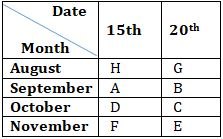S1. Ans. (a)
S2. Ans. (b)
S3. Ans. (d)
S4. Ans. (d)
S5. Ans. (b)

S6. Ans. (c)
Sol.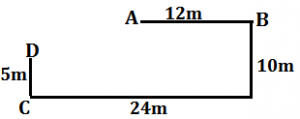Solutions (7-9):
Sol.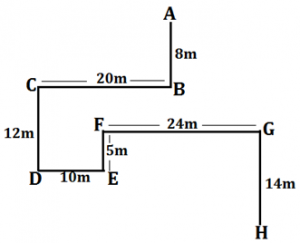S7. Ans. (c)
S8. Ans. (b)
S9. Ans. (d)

S10. Ans. (b)
Sol.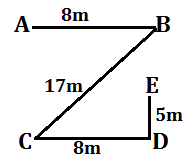S11. Ans. (a)
Sol. I. W<S (True) II. W≤Q (False)

S12. Ans. (d)
Sol. I. E>G (False) II. G>F (False)

S13. Ans. (e)
Sol. I. Y>U (True) II. W≥X (True)

S14. Ans. (d)
Sol. I. B≤D (False) II. L<H (False)

S15. Ans. (c)
Sol. I. P>R (False) II. R=P (False)#### Congratulations!Incorrect details? Fill the form again here

•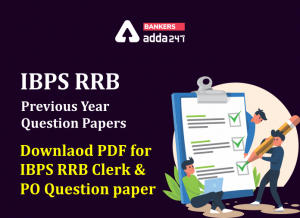IBPS RRB Previous Year Question Paper PD...
•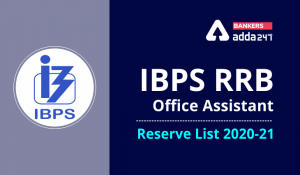IBPS RRB Clerk 2nd Reserve List 2022 Out...
•Reasoning Ability Quiz For IBPS RRB PO C...
•Reasoning Ability Quiz For IBPS Clerk Pr...
•Reasoning Ability Quiz For IBPS RRB PO P...
•Quantitative Aptitude Quiz For IBPS RRB ...